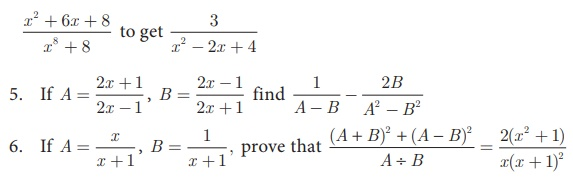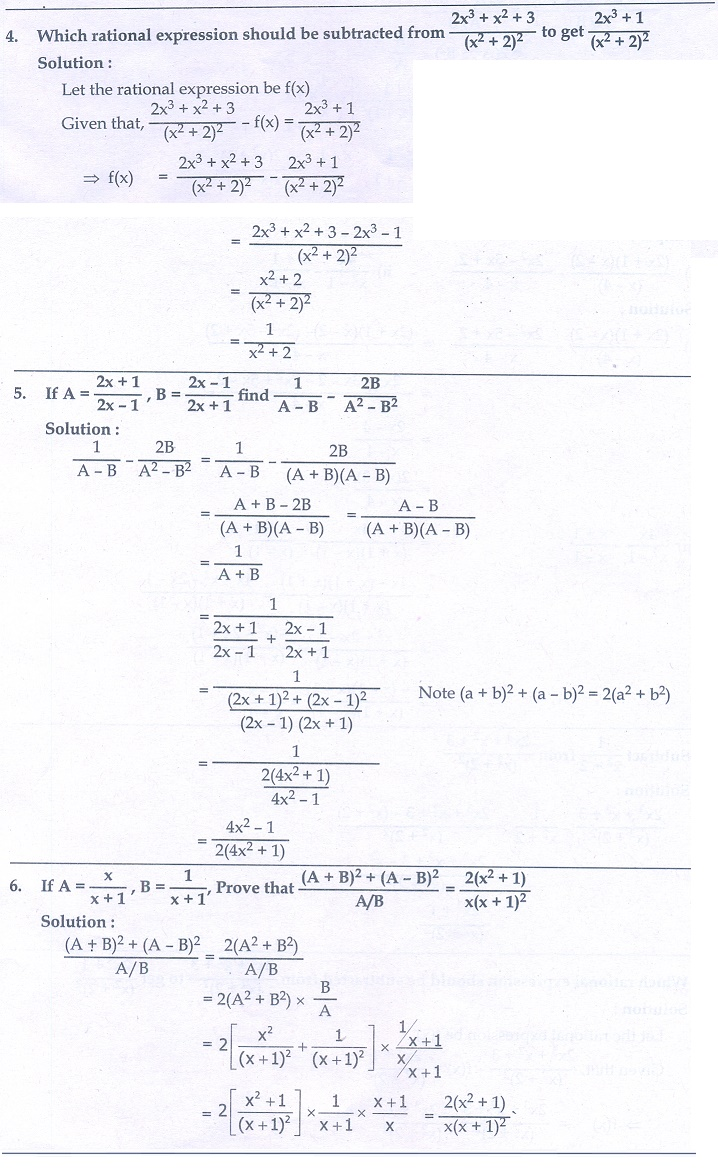Home | | Maths 10th Std | Exercise 3.6: Addition and Subtraction of Rational Expressions

# Exercise 3.6: Addition and Subtraction of Rational Expressions

Maths Book back answers and solution for Exercise questions - Mathematics : Algebra: Operations of Rational Expressions: Addition and Subtraction of Rational Expressions: Exercise Problem Questions with Answer

Exercise 3.64. Which rational expression should be subtracted from7. Pari needs 4 hours to complete a work. His friend Yuvan needs 6 hours to complete the same work. How long will it take to complete if they work together?8. Iniya bought 50 kg of fruits consisting of apples and bananas. She paid twice as much per kg for the apple as she did for the banana. If Iniya bought ₹ 1800 worth of apples and ₹ 600 worth bananas, then how many kgs of each fruit did she buy?